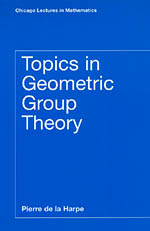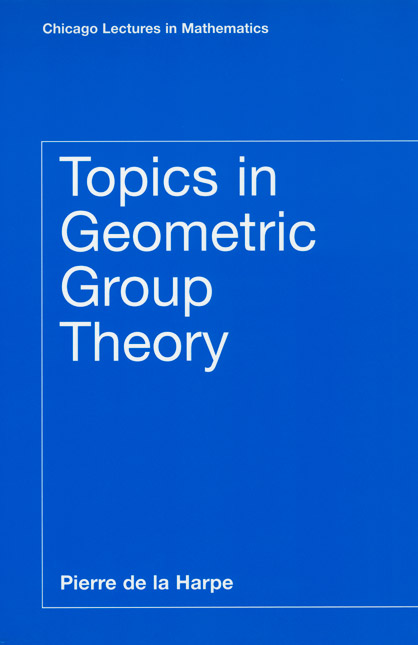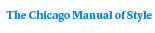# Topics in Geometric Group Theory

Pierre de la HarpeCorrections and updates to the book:
List of January 2003
List of August 2004
List of May 2005
310 pages | 6 x 9 | © 2000
Paper \$41.00 ISBN: 9780226317212 Published September 2000
In this book, Pierre de la Harpe provides a concise and engaging introduction to geometric group theory, a new method for studying infinite groups via their intrinsic geometry that has played a major role in mathematics over the past two decades. A recognized expert in the field, de la Harpe adopts a hands-on approach, illustrating key concepts with numerous concrete examples.

The first five chapters present basic combinatorial and geometric group theory in a unique and refreshing way, with an emphasis on finitely generated versus finitely presented groups. In the final three chapters, de la Harpe discusses new material on the growth of groups, including a detailed treatment of the "Grigorchuk group." Most sections are followed by exercises and a list of problems and complements, enhancing the book’s value for students; problems range from slightly more difficult exercises to open research problems in the field. An extensive list of references directs readers to more advanced results as well as connections with other fields.
Table of Contents• Contents
Contents
Introduction

I. Gauss’ circle problem and Pólya’s random walks on lattices
I.A. The circle problem
I.B. Pólya’s recurrence theorem

II. Free products and free groups
II.A. Free products of groups
II.B. The Table-Tennis Lemma (Klein’s criterion) and examples of free products

III. Finitely-generated groups
III.A. Finitely-generated and infinitely-generated groups
III.B. Uncountably many groups with two generators (B.H. Neumann’s method)
III.C. On groups with two generators
III.D. On finite quotients of the modular group

IV. Finitely-generated groups viewed as metric spaces
IV.A. Word lengths and Cayley graphs
IV.B. Quasi-isometries

V. Finitely-presented groups
V.A. Finitely-presented groups
V.B. The Poincaré theorem on fundamental polygons
V.C. On fundamental groups and curvature in Riemannian geometry
V.D. Complement on Gromov’s hyperbolic groups

VI. Growth of finitely-generated groups
VI.A. Growth functions and growth series of groups
VI.B. Generalities on growth types
VI.C. Exponential growth rate and entropy

VII. Groups of exponential or polynomial growth
VII.A. On groups of exponential growth
VII.B. On uniformly exponential growth
VII.C. On groups of polynomial growth
VII.D. Complement on other kinds of growth

VIII. The first Grigorchuk group
VIII.A. Rooted d-ary trees and their automorphisms
VIII.B. The group r as an answer to one of Burnside’s problems
VIII.C. On some subgroups of r
VIII.D. Congruence subgroups
VIII.E. Word problem and non-existence of finite presentations
VIII.F. Growth
VIII.G. Exercises and complements

References
Index of research problems
Subject index